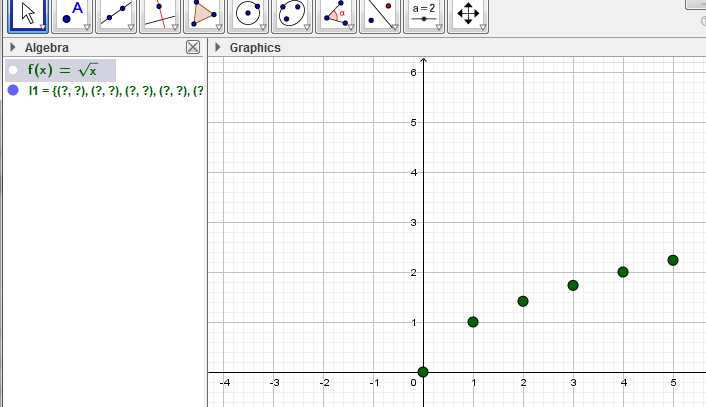# Is that a simply way to define x positive integer ?

anlanz shared this question 1 year ago

Is that a simply way to define x positive integer ?1

yep "x" is reserved for functions

What do you speak about ? Do you want, for example, define a function with x positive integer..? or..?1

I mean the graph only shows points where Xs are positive integers in a function, instead of a curve.1

Some graphs are really showing slow, hard to move or zoom in zoom out. They really need to improve the algorithms.1

Perhaps you can put the function in an if-clause

if(x>0, x^2-x-1)

so what?1

something like that?1

sequence((n,f(n)),n,floor(x(corner(1))),ceil(x(corner(2))))

hide f1

Thanks. it works, but still has problems that I cannot turn the curve off, if turn it off, the points are gone. Another problem is could i just pick points f(x)> 0 to be shown? Thanks a lot.1

"is could i just pick points f(x)> 0 to be shown?"

KeepIf(y(A)>0, A,sequence((n,f(n)),n,floor(x(corner(1))),ceil(x(corner(2)))))1